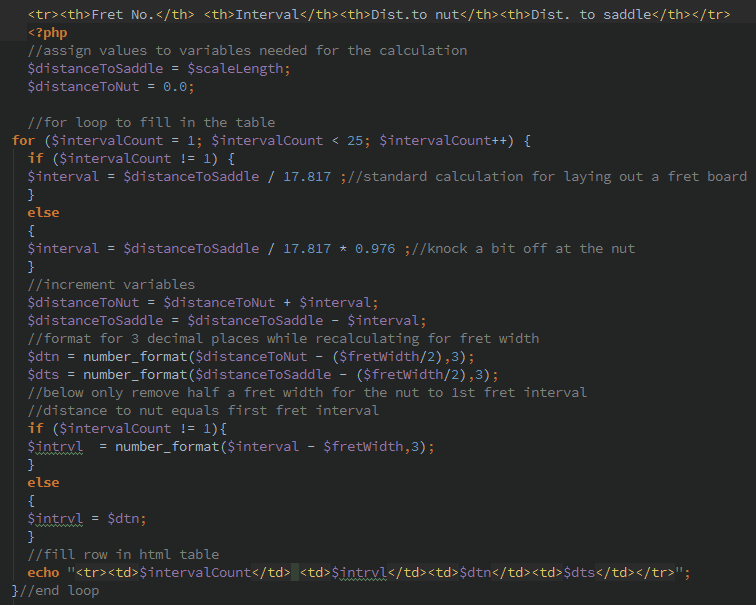## Understanding The Fret Calculator

The strings drive the sound on a guitar. The points of contact between the string and the guitar are: the nut, the saddle and the frets. When a guitar is in operating condition, the fretboard and points of contact are a complex curved plane that is constantly moving. When a guitar is being built or repaired the fretboard should be static, in the form of a plane, cylinder or cone section with under 0.001" tolerance. The luthier's goal is to measure and cut the fret slots then install the frets such that there are no out of tune notes on the guitar. Let it be said that this is an impossible task and the guitar players skill and hearing are required to make the very best guitar play in tune. However, it is the luthier's responsibility to minimize the guitar player's effort by placing the nut, saddle and frets properly.

The fret calculator can be used to layout a fretboard or make a jig for repeated use. Additionally, the fret calculator can be used to measure and analyze intonation problems on any fretted instrument. A function has been added to input the width of a fret. If this function is desired, the user can measure a fret then enter its width in the fret calculator and the output can be used to compare measurements from the fret edges. Otherwise, to layout a fingerboard or jig, enter 0 for the fret width.

Scale length is the baseline measurement for the length of the strings on a guitar. For many popular instruments this will be published on the builder's or other websites. The scale length can also be measured from nut to saddle with a ruler or tape measure. Keep in mind that saddles may be compensated or misplaced by the builder. Generally, the scale length is twice the distance from the nut to the 12th fret. We can safely assume the builder has used a round number in millimeters; or inches and a limited amount of decimal places. Some experimentation may be needed to get an accurate measured scale length.

Fret width should be measured with a caliper at the base of the fret. Additionally, fret manufacturers and suppliers can provide this information if the model number or style of the fret is known.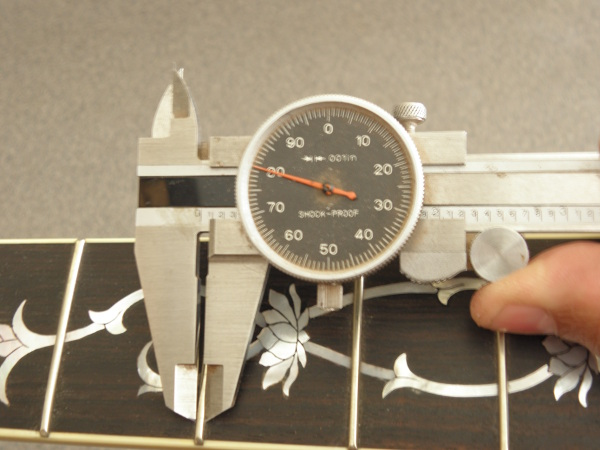Figure 1: Measuring fret width

This fret measures 0.080". Enter 0.080 or .08 in the fret calculator for fret width. Make sure the fret width and scale length are of the same measure: e.g., inches and inches or millimeters and millimeters.

Fret Number defines which fret or fret slot will be the measuring point; starting from the nut or zero fret and counting towards the saddle.

Interval represents the distance between the subject fret (fret number) and the fret previously counted; i.e, The third fret interval is the distance between the third and second frets.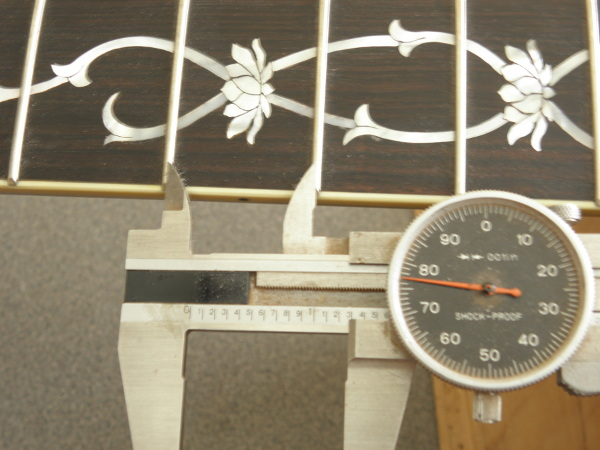Figure 2: Measuring fret interval

For a 25.5" scale length the third fret interval will be displayed as 1.277. Looking at figure 2, we can see that it may be difficult to test this measurement by setting the caliper points on a polished convex surface. The fret calculator allows for fret widths to be input so accurate measurements can be made at the base of the fret; i.e., the caliper points can bump against a hard surface (figure 3).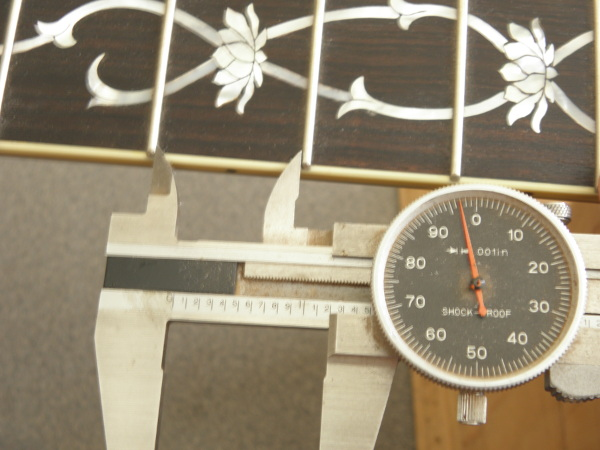Figure 3: Measuring 3rd fret interval

Distance to nut displays the distance between the fret and the nut. This is the measurement for laying out a fingerboard or jig. Important: The fret calculator adds a compensation at the nut which is not used by most manufacturers. The first fret interval is shortened by 2.4% during the calculation to compensate for the string height at the nut being slightly higher than the frets. This has been tested in over 200 instruments and by top level musicians in the most discerning environments.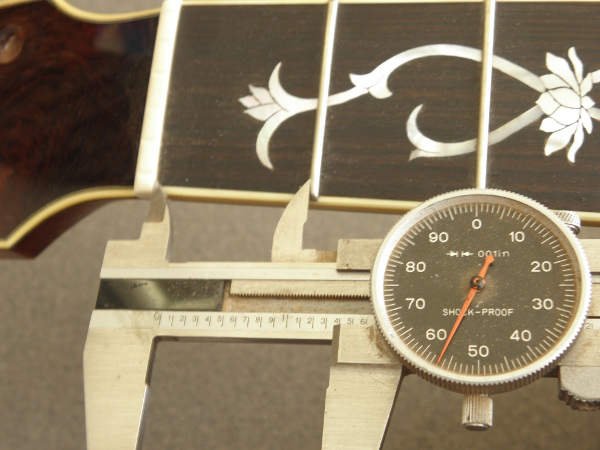Figure 4: Measuring 1st fret interval

For a 25.5" Scale length, the uncompensated first fret to nut measurement would be displayed as 1.431. The fret calculator multiplies this number by 0.976 for the string height compensation then subtracts half of a fret's width since the nut's face is placed on the measuring line, not straddling it like a fret. Thus, ((25.5 / 17.817) x 0.976) - (0.080 /2) = 1.356 which is the measurement illustrated in figure 4. In figure 5, measuring from the third fret, the compensation is still included. However, the 0.034" taken off at the nut creates a 5 cent difference in the note played on that fret. Differences of less than 4 cents are unrecognized by normal humans. In other words, the nut compensation effects are encapsulated in the first position where most people play and unnoticed after the third or fourth fret.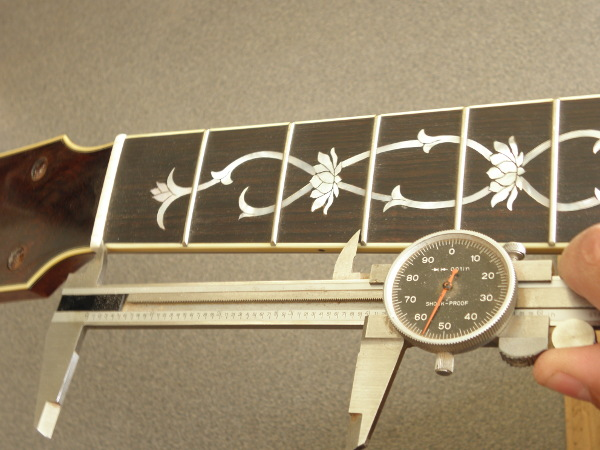Figure 4: Measuring interval between nut and 3rd fret

Distance to saddle is the remainder after each fret interval is decremented. A special device such as an extra long vernier caliper or CNC machine with special tools is needed to measure this accurately. With this in mind, distance to saddle is included to fulfill the needs of students or scientists interested in guitars.

Equal temperament is a method of measuring 12 equivalent intervals to the octave. Based on the 12th root of two discovered by Zhu Zaiyu in 1584, it determines the baseline intervals in music. It would look like this as a word problem in mathematics:

frequency * 2^(1/12) = next frequency

Using musical notes and their frequencies it would look like this:

A 440hz * 2^(1/12) = A# or Bb 466.164hz

With the fret calculator, we are shortening the distance to the saddle each time we calculate a fret interval. This equation determines how much a given scale length needs to be shortened to increment one musical note; e.g., A to A# or Bb:

distance to nut = scale length - ( scale length / (2^( 1/12 ))

For the first fret interval, the scale length equals the distance to the saddle. Subsequent fret intervals would use:

distance to nut (at next fret) = distance to saddle - ( distance to saddle / (2^( 1/12 ))

also: distance to nut at fret number = scale length - ( scale length / (2^( fret number/12 ))

For example: 1.431 = 25.5 - (25.5 / (2^(1/12))) , 1.277 = 22.750 - ( 22.75 / (2^(1/12))) and 4.057 = 25.5 - ( 25.5 / (2^(3/12))) Keep in mind the nut compensation and rounding

Applying the twelfth root of two works well in computers but for calculations by hand accuracy will suffer if there are not enough decimal places. If we divide the scale length by the first fret interval we get a common divisor: 25.5/1.4312 =17.817. The fret calculator uses this divisor to complete the table. It makes the code easier to read, requirements for accuracy are met and skeptics can double check calculations by hand or with their smart phone.

The calculation looks like this: